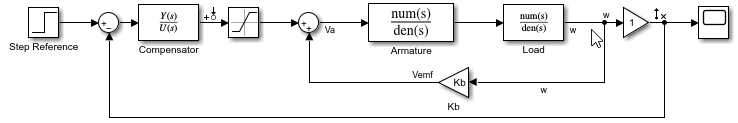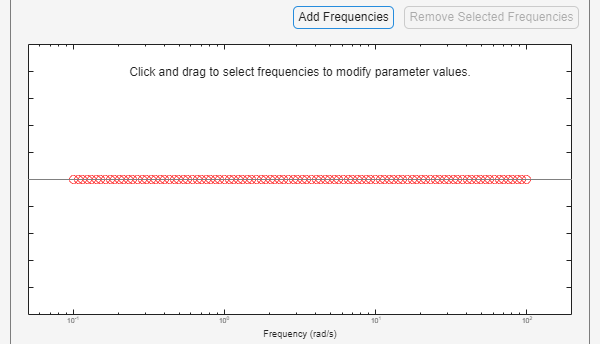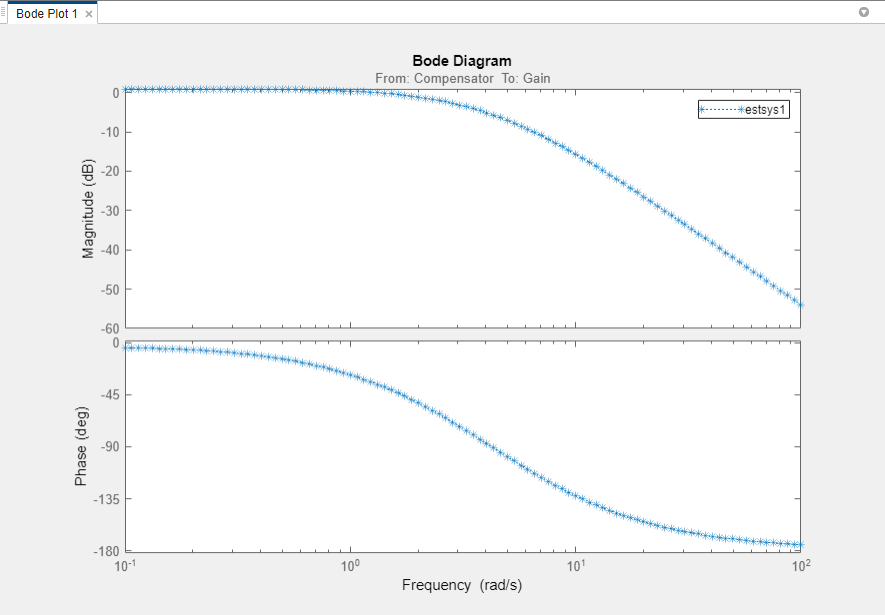## Estimate Frequency Response Using Model Linearizer

This example shows how to estimate the frequency response of a portion of a Simulink® model using the Model Linearizer. To estimate the frequency response, you specify the portion of the model you want to estimate, the operating point for estimation, and the input signal to use for estimation.

### Open Simulink Model and Model Linearizer

```sys = 'scdDCMotor'; open_system(sys)```To open the Model Linearizer, in the Simulink model window, in the Apps gallery, click Model Linearizer.

### Specify Portion of Model to Estimate

By default, Model Linearizer uses the linearization analysis points defined in the model (the model I/Os) to determine where to inject the test signal and where to measure the frequency response. The model `scdDCMotor` contains predefined linear analysis points: an input point at the compensator output, and an open-loop output after the unit gain block. For this example, use these predefined model I/Os to obtain the frequency response of the inner loop of the model with the outer loop open.

If you want to obtain the frequency response of a different portion of the model, in the Estimation tab of Model Linearizer, use the drop-down list. (Analysis points for estimation work the same way as analysis points for linearization. For more information about linear analysis points, see Specify Portion of Model to Linearize.)

### Specify Operating Point for Estimation

You perform frequency response estimation at a steady-state operating point of the model. You can compute or specify an operating point in Model Linearizer using the drop-down list. By default, Model Linearizer uses the operating point defined by the model initial conditions. For this example, use that operating point. (For more information about operating points, see About Operating Points.)

### Create Input Signal for Estimation

Frequency response estimation injects an input signal into the input analysis point you specify for estimation. For this example, configure a sinestream signal, which is a series of sinusoidal perturbations at frequencies you specify. (For more information about input signals, see Estimation Input Signals.)

1. On the Estimation tab, in the Input Signal drop-down list, select `Sinestream`. The Create sinestream input dialog box opens.2. To specify frequency points for the input signal, click. In the Add frequencies dialog box, specify the frequency range and number of points for the input signal. The frequency points you specify are the frequencies at which Model Linearizer computes the estimated response.

For this example, specify a range from 0.1 to 100 rad/s. Also, specify 100 logarithmically spaced frequencies.Click OK. The added points are visible in the frequency content viewer of the Create sinestream input dialog box.Select all these frequency points for the estimation input signal.3. Specify the amplitude of the input signal. Enter `1` in the Amplitude field. When you specify a scalar value, Model Linearizer uses the same amplitude for all frequencies.

4. Click to create the sinestream input signal. The new input signal, `in_sine1`, appears in the Linear Analysis Workspace.

### Estimate Frequency Response

You can now estimate the frequency response and generate a frequency-domain plot of the result. To do so, clickBode. The estimated frequency response, appears in the Linear Analysis Workspace as the `frd` model `estsys1`.To export the estimated frequency response model to the MATLAB® workspace for further analysis, drag it from the Linear Analysis Workspace section of the Data Browser to the MATLAB Workspace section.### Analyze Estimated Frequency Response

The simulation results viewer in Model Linearizer lets you examine further details of the frequency response estimation. For more information, see Analyze Estimated Frequency Response.

## Related Topics